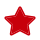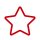# 地理关系函数

#### st_area

`Double st_area(Geometry g)`

• 查询命令：

select st_area(st_geomFromWKT(location)) from geotbl where id='1'

• 查询结果：
`550.0`

#### st_centroid

`Point st_centroid(Geometry g)`

• 查询命令：

select st_asText(st_centroid(st_geomFromWKT(location))) from geotbl where id='4'

• 查询结果：
`POINT (27.40740740740741 28.765432098765434)`

#### st_closestPoint

`Point st_closestPoint(Geometry a, Geometry b)`

• 查询命令：

select st_asText(st_closestPoint((select st_geomFromWKT(location) from geotbl where id='1'),(select st_geomFromWKT(location) from geotbl where id='2')))

• 查询结果：
`POINT (40 40)`

#### st_contains

`Boolean st_contains(Geometry a, Geometry b)`

• 查询命令：

select st_contains((select st_geomFromWKT(location) from geotbl where id='1'),(select st_geomFromWKT(location) from geotbl where id='2'))

• 查询结果：
`FALSE`

#### st_covers

`Boolean st_covers(Geometry a, Geometry b)`

• 查询命令：

select st_covers((select st_geomFromWKT(location) from geotbl where id='1'),(select st_geomFromWKT(location) from geotbl where id='2'))

• 查询结果：
`FALSE`

#### st_crosses

`Boolean st_crosses(Geometry a, Geometry b)`

• 查询命令：

select st_crosses((select st_geomFromWKT(location) from geotbl where id='1'),(select st_geomFromWKT(location) from geotbl where id='2'))

• 查询结果：
`FALSE`

#### st_disjoint

`Boolean st_disjoint(Geometry a, Geometry b)`

• 查询命令：

select st_disjoint((select st_geomFromWKT(location) from geotbl where id='1'),(select st_geomFromWKT(location) from geotbl where id='2'))

• 查询结果：
`TRUE`

#### st_distance

`Double st_distance(Geometry a, Geometry b)`

• 查询命令：

select st_distance((select st_geomFromWKT(location) from geotbl where id='1'),(select st_geomFromWKT(location) from geotbl where id='2'))

• 查询结果：
`25.4950975679639`

#### st_distanceSphere

`Double st_distanceSphere(Geometry a, Geometry b)`

• 查询命令：

select st_distanceSphere((select st_geomFromWKT(location) from geotbl where id='1'),(select st_geomFromWKT(location) from geotbl where id='2'))

• 查询结果：
`3284010.93490738`

#### st_distanceSpheroid

`Double st_distanceSpheroid(Geometry a, Geometry b)`

• 查询命令：

select st_distanceSpheroid((select st_geomFromWKT(location) from geotbl where id='1'),(select st_geomFromWKT(location) from geotbl where id='2'))

• 查询结果：
`3288016.93279476`

#### st_equals

`Boolean st_equals(Geometry a, Geometry b)`

• 查询命令：

select st_equals((select st_geomFromWKT(location) from geotbl where id='1'),(select st_geomFromWKT(location) from geotbl where id='2'))

• 查询结果：
`FALSE`

#### st_intersects

`Boolean st_intersects(Geometry a, Geometry b)`

• 查询命令：

select st_intersects((select st_geomFromWKT(location) from geotbl where id='1'),(select st_geomFromWKT(location) from geotbl where id='2'))

• 查询结果：
`FALSE`

#### st_length

`Double st_length(Geometry geom)`

• 查询命令：

select st_length(st_geomFromWKT(location)) from geotbl where id='4'

• 查询结果：
`160.12062648706927`

#### st_lengthSphere

`Double st_lengthSphere(LineString line)`

• 查询命令：

select st_lengthSphere(st_castToLineString(st_geomFromWKT(location))) from geotbl where id='5'

• 查询结果：
`1.0013773137296816E7`

#### st_lengthSpheroid

`Double st_lengthSpheroid(LineString line)`

• 查询命令：

select st_lengthSpheroid(st_castToLineString(st_geomFromWKT(location))) from geotbl where id='5'

• 查询结果：
`1.0001465052274073E7`

#### st_overlaps

`Boolean st_overlaps(Geometry a, Geometry b)`

• 查询命令：

select st_overlaps((select st_geomFromWKT(location) from geotbl where id='1'),(select st_geomFromWKT(location) from geotbl where id='2'))

• 查询结果：
`FALSE`

#### st_relate

`String st_relate(Geometry a, Geometry b)`

• 查询命令：

select st_relate((select st_geomFromWKT(location) from geotbl where id='1'),(select st_geomFromWKT(location) from geotbl where id='2'))

• 查询结果：
`FF2FF1212`

#### st_relateBool

`Boolean st_relateBool(Geometry a, Geometry b, String mask)`

• 查询命令：

select st_relateBool((select st_geomFromWKT(location) from geotbl where id='1'),(select st_geomFromWKT(location) from geotbl where id='2'),'FF2FF1212')

• 查询结果：
`TRUE`

#### st_touches

`Boolean st_touches(Geometry a, Geometry b)`

• 查询命令：

select st_touches((select st_geomFromWKT(location) from geotbl where id='1'),(select st_geomFromWKT(location) from geotbl where id='2'))

• 查询结果：
`FALSE`

#### st_within

`Boolean st_within(Geometry a, Geometry b)`

• 查询命令：

select st_within((select st_geomFromWKT(location) from geotbl where id='1'),(select st_geomFromWKT(location) from geotbl where id='2'))

• 查询结果：
`FALSE`

# 相关产品